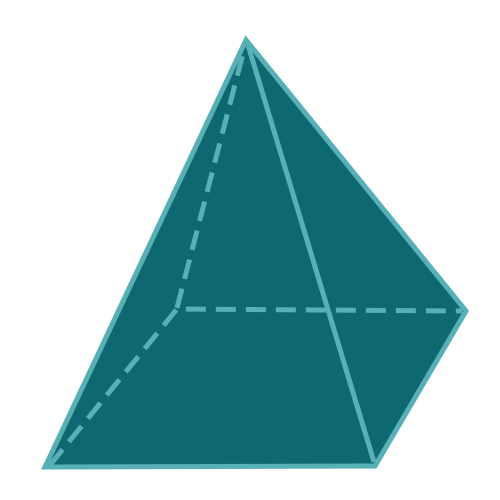Report a Problem
Suggestions

#Calculate The Surface Area of A Pyramid With A Square Base

Last updated: Saturday, April 29, 2023
Select a type of pyramid below
Rectangular Base
Square Base
Equilateral Triangular Base
Tetrahedron
Pentagonal Base
Hexagonal Base

A pyramid with a square base is a fascinating three-dimensional geometric figure characterized by a flat, square base connected to a single apex point by four congruent triangular lateral faces. This captivating structure has inspired numerous applications in mathematics, physics, and science, as well as engineering and architecture. Within the context of surface area, the concept refers to the total area covering the external faces of the pyramid, which is of great importance for understanding its properties and practical applications.

In mathematics, the study of pyramids with square bases provides insight into the properties of three-dimensional shapes, solid geometry, and the relationships between different types of polygons. These concepts form the foundation for a deeper understanding of geometry and its real-world applications. In physics, the surface area of a pyramid with a square base can be used to analyze and predict properties such as center of mass, stability, and stress distribution, which are vital for the design and construction of structures and objects.

In science, the study of such pyramids has contributed to the development of crystallography, where the geometry of crystal structures and their surface area properties are essential for understanding the arrangement of atoms, ions, or molecules. This knowledge is crucial for advancements in material science, pharmaceuticals, and nanotechnology. In architecture, pyramids with square bases have inspired the design of iconic structures, such as the Great Pyramid of Giza in Egypt, showcasing the combination of aesthetics, functionality, and historical significance.

The surface area of a pyramid with a square base plays a critical role in these applications, as it directly impacts the understanding of its properties and practical implications.

The formula for determining the surface area of a pyramid with a square base is defined as:
$$SA$$ $$=$$ $$a^2$$ $$+$$ $$a$$ $$\cdot$$ $$\sqrt{a^2 + 4 \cdot h^2}$$
$$SA$$: the surface area of the pyramid
$$a$$: the length of of any side of the base
$$h$$: the height of the pyramid
The SI unit of surface area is: $$square \text{ } meter\text{ }(m^2)$$

## Find $$SA$$

Use this calculator to determine the surface area of a pyramid with square base using the base area and the height.
Hold & Drag
CLOSE
the length of of any side of the base
$$a$$
$$meter$$
the height of the pyramid
$$h$$
$$meter$$
Bookmark this page or risk going on a digital treasure hunt again